# ３１　Entanglement Entropy #gravity #universe #QuantumTheory #SuperStringTheory

３１　Entanglement Entropy #gravity #universe #QuantumTheory #SuperStringTheory

○ Quantum Entanglement Entropy(EE)：Number of quantum entanglements. An indicator of the quantification of quantum entanglement. = Number of EPR pair (EPR: Einstein-Podolsky-Rosen)

○ It is very difficult to calculate the entropy of quantum with quantum field theory, but in Anti de Sitter space(Ads), like a holography, It can be replaced by calculation of surface area of a bubble (like a black hole with the smallest surface area). ：Ryu-Takayanagi formula(Holographic Entanglement Entropy Formula 2006) 

※Anti de Sitter space(Ads)：One of the spaces where the curvature is negative and constant
= Universe with negative cosmic constant Λ (dark energy)
⇔de Sitter space(dS) (Positive curvature)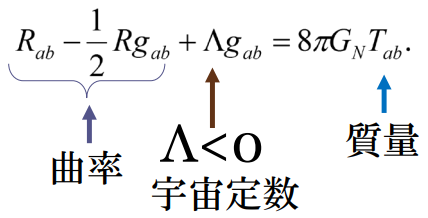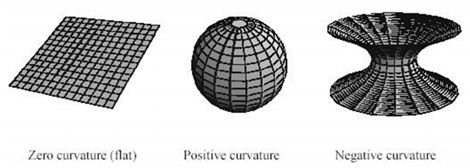Figure：Curvature 

• A major feature of AdS is to be able to cover infinite space in a finite area.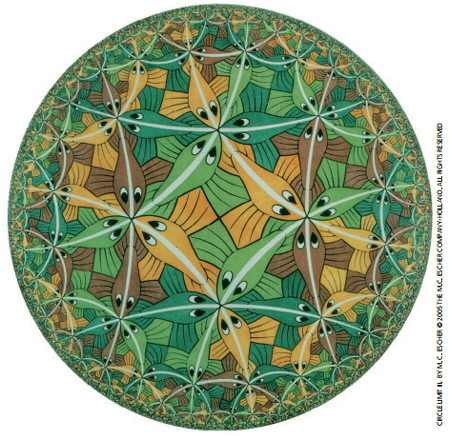Firure：Escher's picture: It is close to the image which projected Anti de Sitter space on the plane.

• Although de Sitter space universe (Λ> 0) is close to the real universe, its holographic principle has not been elucidated. 

○Ryu-Takayanagi formula：Holographic Entanglement Entropy Formula (2006)
• They revealed that holographic principle is related to quantum entanglement.
• Calculation of the entropy of quantum entanglement in quantum field theory can be replaced with calculation of the surface area of bubble (shaped to minimize the surface area) generated in AdS.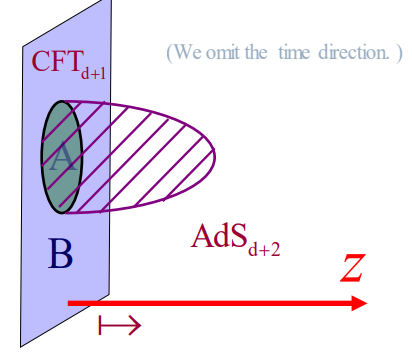Figure：Thinking of the minimal aspect extending from the interface to the inside of the AdS space, calculating its area and dividing it by 4 times the Newton's constant of gravity theory is equivalent to entanglement entropy.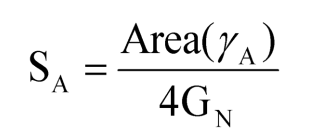※Ryu-Takayanagi formula：Holographic Entanglement Entropy Formula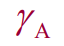is the minimal area surface
・Generalization of Bekenstein-Hawking entropy formula.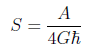※Bekenstein-Hawking entropy 

○In Ryu-Takayanagi formula, one bit of quantum entanglement is present per planck area (1 square planck length).

※Planck length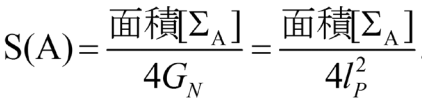○Task：It is necessary to elucidate the quantum entanglement in de Sitter space.

○Application of quantum entangy entropy:
・Entangy entropy (EE) is helpful for understanding s of various (quantum) gravity phenomena such as black hole formations, singularities etc. 

・In certain substances, controlling interatomic distances and temperatures leads to the transition to "strange metal" (anomalous metallic phase), which has different electrical characteristics (superconductivity) from ordinary metals, It is caused by becoming quantum entangled state as a whole (rapid increase of quantum entanglement entropy).

【Refereces】
1. Ryu-Takayanagi Conjecture - Wikipedia
2. T. Takayanagi" Front line of super string theory：Is the universe created from qubits？“ 20170524(Japanese)